# Graphs with four or fewer vertices

Relevant articles: , , , , , .

[hide]

# Graphs with four or fewer vertices

There are ten non-isomorphic graphs with four or fewer vertices, excluding those with isolated vertices, and these are shown in the Figure 1.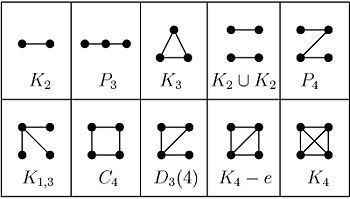## Spectrum Results

The spectrum problem is completely settled for graphs all with up to four vertices, excluding those with isolated vertices, and Table 1 summarises these results. An explanation of the sources of these results is given in .

Table 1

 Graph Spectrum$K_{2}$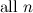${\rm all \ }n$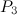$P_{3}$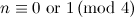$n\equiv 0{\rm \ or \ } 1 \,({\rm mod \ }4)$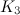$K_{3}$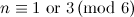$n\equiv 1{\rm \ or \ } 3 \,({\rm mod \ }6)$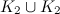$K_2 \cup K_2$$n\equiv 0{\rm \ or \ } 1 \,({\rm mod \ }4)$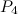$P_{4}$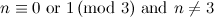$n\equiv 0{\rm \ or \ } 1 \,({\rm mod \ }3){\rm \ and \ }n\neq 3$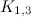$K_{1,3}$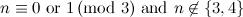$n\equiv 0{\rm \ or \ } 1 \,({\rm mod \ }3){\rm \ and \ }n\not\in\{3,4\}$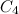$C_{4}$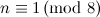$n\equiv 1 \,({\rm mod \ }8)$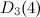$D_{3}(4)$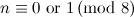$n\equiv 0{\rm \ or \ } 1 \,({\rm mod \ }8)$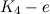$K_{4} - e$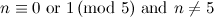$n\equiv 0{\rm \ or \ } 1 \,({\rm mod \ }5){\rm \ and \ }n\neq 5$$K_{4}$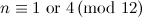$n\equiv 1{\rm \ or \ } 4 \,({\rm mod \ }12)$

## Notes

• The complete graphs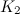$K_2$,$K_3$ and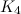$K_4$ are discussed in the section on complete graphs.
• The spectrum problems for the path$P_3$ and the graph$K_2\cup K_2$ are easy exercises, and their solutions are covered by Theorem 1 in the section on Paths and Theorem 1 in the section on Matchings.
• A solution for$P_4$ is also given by Theorem 1 in the section on Paths.
• Note that$K_4-e$ is a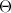$\Theta$-graph.
• Also note that$D_3(4)$ is a dragon.

## References

1. 1.0 1.1 Adams, P., Bryant, D., and Buchanan, M. A survey on the existence of G-designs, J. Combin. Des. 16, 373–410 (2008).
2. Bermond, J. -C. Cycles dans les graphes et G-configurations, PhD Thesis (1975).
3. Bermond, J. -C. and Schönheim, J. \$G-decomposition of K_n, where G has four vertices or less, Discrete Math. 19, 113–120 (1977).
4. Cain, P. Decomposition of complete graphs into stars, Bull. Austral. Math. Soc. 10, 23–30 (1974).
5. Kotzig, A. Decomposition of a complete graph into 4k-gons, Mat.-Fyz. u Casopis Sloven. Akad. Vied, 15, 229–233 (1965).
6. Tarsi, M. Decomposition of a complete multigraph into simple paths: nonbalanced handcuffed designs, J. Combin. Theory Ser. A, 34, 60–70 (1983).